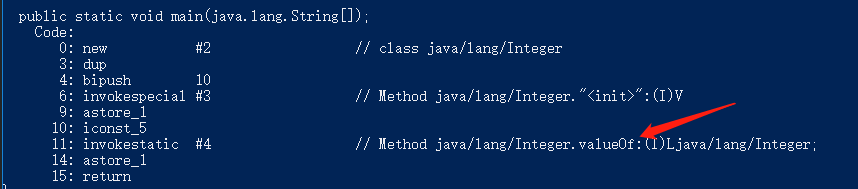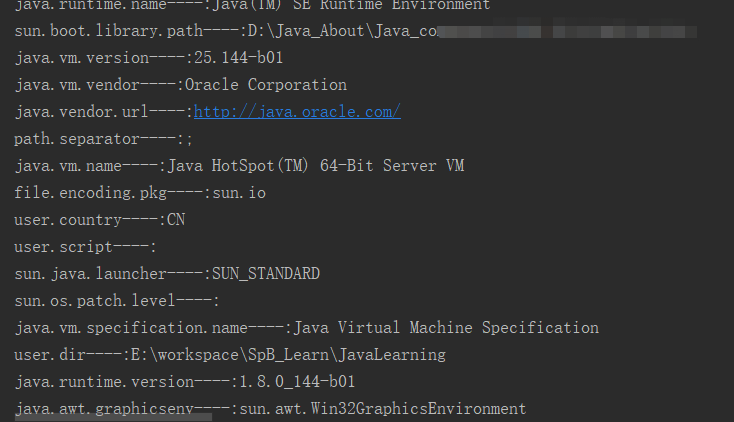# Java之Integer类源码学习

hresh 394### 类定义

public final class Integer extends Number implements Comparable<Integer>


1、Integer 类不能被继承(其他包装类也都是 final 修饰的，不可被继承)

2、Integer 类实现了 Comparable 接口，所以可以用 compareTo 进行比较并且 Integer 对象只能和
Integer 类型的对象进行比较，不能和其他类型比较（至少调用 compareTo 方法无法比较）。

3、Integer 继承了 Number 类，所以该类可以调用
longValue、floatValue、doubleValue等系列方法返回对应的类型的值。

### 属性

#### 1、私有属性

Integer 类中定义了以下几个私有属性：

private final int value;
private static final long serialVersionUID = 1360826667806852920L;


serialVersionUID 和序列化有关。String 的源码学习中有介绍，这里不再赘述。

public Integer(int value) {
this.value = value;
}


public class IntegerTest {
public static void main(String[] args) {
Integer i = new Integer(10);
i = 5;
}
}#### 2、公共属性

//值为 （－（2的31次方）） 的常量，它表示 int 类型能够表示的最小值。
public static final int MIN_VALUE = -2147483648;
//值为 （（2的31次方）－1） 的常量，它表示 int 类型能够表示的最大值。
public static final int MAX_VALUE = 2147483647;
//表示基本类型 int 的 Class 实例。
public static final Class<Integer> TYPE = Class.getPrimitiveClass("int");
static final char[] digits = new char[]{'0', '1', '2', '3', '4', '5', '6', '7', '8', '9', 'a', 'b', 'c', 'd', 'e', 'f', 'g', 'h', 'i', 'j', 'k', 'l', 'm', 'n', 'o', 'p', 'q', 'r', 's', 't', 'u', 'v', 'w', 'x', 'y', 'z'};
static final char[] DigitTens = new char[]{'0', '0', '0', '0', '0', '0', '0', '0', '0', '0', '1', '1', '1', '1', '1', '1', '1', '1', '1', '1', '2', '2', '2', '2', '2', '2', '2', '2', '2', '2', '3', '3', '3', '3', '3', '3', '3', '3', '3', '3', '4', '4', '4', '4', '4', '4', '4', '4', '4', '4', '5', '5', '5', '5', '5', '5', '5', '5', '5', '5', '6', '6', '6', '6', '6', '6', '6', '6', '6', '6', '7', '7', '7', '7', '7', '7', '7', '7', '7', '7', '8', '8', '8', '8', '8', '8', '8', '8', '8', '8', '9', '9', '9', '9', '9', '9', '9', '9', '9', '9'};
static final char[] DigitOnes = new char[]{'0', '1', '2', '3', '4', '5', '6', '7', '8', '9', '0', '1', '2', '3', '4', '5', '6', '7', '8', '9', '0', '1', '2', '3', '4', '5', '6', '7', '8', '9', '0', '1', '2', '3', '4', '5', '6', '7', '8', '9', '0', '1', '2', '3', '4', '5', '6', '7', '8', '9', '0', '1', '2', '3', '4', '5', '6', '7', '8', '9', '0', '1', '2', '3', '4', '5', '6', '7', '8', '9', '0', '1', '2', '3', '4', '5', '6', '7', '8', '9', '0', '1', '2', '3', '4', '5', '6', '7', '8', '9', '0', '1', '2', '3', '4', '5', '6', '7', '8', '9'};
static final int[] sizeTable = new int[]{9, 99, 999, 9999, 99999, 999999, 9999999, 99999999, 999999999, 2147483647};
//用来以二进制补码形式表示 int 值的比特位数。
public static final int SIZE = 32;
//int的字节数
public static final int BYTES = 4;


### 方法

#### 构造方法

Integer 提供了两个构造方法：

public Integer(int var1) {
this.value = var1;
}

public Integer(String var1) throws NumberFormatException {
this.value = parseInt(var1, 10);
}


#### Integer valueOf()方法

valueOf()方法被声明为静态方法，共有三种实现方式。

public static Integer valueOf(String var0, int var1) throws NumberFormatException {
return parseInt(var0, var1);
}

public static Integer valueOf(String var0) throws NumberFormatException {
return parseInt(var0, 10);
}

public static Integer valueOf(int var0) {
return var0 >= -128 && var0 <= Integer.IntegerCache.high ? Integer.IntegerCache.cache[var0 + 128] : new Integer(var0);
}


Integer i = 100;//转换为Integer i = Integer.valueOf(100)
Integer j = 100;
Integer ii = 128;//转换为Integer ii = Integer.valueOf(128)
Integer jj = 128;

//执行结果为：


private static class IntegerCache {
static final int low = -128;
static final int high;
static final Integer[] cache;

private IntegerCache() {
}

static {
int var0 = 127;
String var1 = VM.getSavedProperty("java.lang.Integer.IntegerCache.high");
int var2;
if (var1 != null) {
try {
var2 = Integer.parseInt(var1);
var2 = Math.max(var2, 127);
var0 = Math.min(var2, 2147483518);
} catch (NumberFormatException var4) {
}
}

high = var0;
cache = new Integer[high - -128 + 1];
var2 = -128;

for(int var3 = 0; var3 < cache.length; ++var3) {
cache[var3] = new Integer(var2++);
}

assert high >= 127;

}
}


//当传入的字符串含有中英文字符时，会提示报错。目前测试仅支持数字字符串，不包括小数点，可以包含正负符号
public static int parseInt(String var0, int var1) throws NumberFormatException {//var1为10
if (var0 == null) {
throw new NumberFormatException("null");
} else if (var1 < 2) {
} else if (var1 > 36) {
} else {
int var2 = 0;
boolean var3 = false;
int var4 = 0;
int var5 = var0.length();
int var6 = -2147483647;
if (var5 > 0) {
char var9 = var0.charAt(0);//获取字符串首字符，确定最后结果的正负
if (var9 < '0') {//Character.hashCode('0')等于48，'-'和'+'均小于它
if (var9 == '-') {//假如var0为“-1234”时，当首字符为'-'，最后结果也为负值，否则都为正值
var3 = true;
var6 = -2147483648;
} else if (var9 != '+') {
throw NumberFormatException.forInputString(var0);
}

if (var5 == 1) {
throw NumberFormatException.forInputString(var0);
}

++var4;
}

int var8;
for(int var7 = var6 / var1; var4 < var5; var2 -= var8) {
//在指定的基数返回字符ch的数值,在此程序中基数为十进制，若为中文字符，返回为-1
var8 = Character.digit(var0.charAt(var4++), var1);
if (var8 < 0) {
throw NumberFormatException.forInputString(var0);
}

if (var2 < var7) {
throw NumberFormatException.forInputString(var0);
}

var2 *= var1;
if (var2 < var6 + var8) {
throw NumberFormatException.forInputString(var0);
}
}

return var3 ? var2 : -var2;
} else {
throw NumberFormatException.forInputString(var0);
}
}
}

public static int parseInt(String var0) throws NumberFormatException {
return parseInt(var0, 10);
}


public static void parseInt(String var0,int var1){
System.out.println(Integer.parseInt(var0,var1));
}

parseInt("0", 10); //返回 0
parseInt("473", 10); //返回 473
parseInt("-0", 10);// 返回 0
parseInt("+0", 10);// 返回 0
parseInt("12345",16);//74565
parseInt("acbe",16);//44222
parseInt("-FF", 16);// 返回 -255
parseInt("1100110", 2);// 返回 102
parseInt("2147483647", 10);// 返回 2147483647
parseInt("-2147483648", 10);// 返回 -2147483648
parseInt("2147483648", 10);// 抛出 NumberFormatException
parseInt("77", 8);// 返回 63
parseInt("99", 8);// 抛出 NumberFormatException
parseInt("Hollis", 10);// 抛出 NumberFormatException
parseInt("Hollis", 27);// 抛出 NumberFormatException


#### String 转成 Integer（int）的方法

Integer getInteger(String nm)
Integer getInteger(String nm, int val)
Integer getInteger(String nm, Integer val)
Integer decode(String nm)
Integer valueOf(String s)
int parseUnsignedInt(String s)
int parseInt(String s)


getInteger(String nm) ---> getInteger(nm, null);--->Integer.decode()--->Integer.valueOf()--->parseInt()

##### 1、getInteger()方法
public static Integer getInteger(String var0, Integer var1) {
String var2 = null;

try {
var2 = System.getProperty(var0);
} catch (NullPointerException | IllegalArgumentException var4) {
}

if (var2 != null) {
try {
return decode(var2);
} catch (NumberFormatException var5) {
}
}

return var1;
}


Properties props = System.getProperties();
Set<Object> set = props.keySet();
for(Object obj:set){
System.out.println(String.format("%s----:%s",obj.toString(),props.getProperty(obj.toString())));
}
props.put("hollis.integer.test.key","10000");
Integer i = Integer.getInteger("hollis.integer.test.key");
System.out.println(i);
//输出 10000getInteger(String nm,int val)
getInteger(String nm)

System.out.println(Integer.getInteger("hello"));//null
System.out.println(Integer.getInteger("hello",10));//输出10
System.out.println(Integer.getInteger("",10));//输出10
System.out.println(Integer.getInteger(null,10));//输出10


##### 2、decode()方法
//将 String 解码为 Integer。接受十进制、十六进制和八进制数字
public static Integer decode(String var0) throws NumberFormatException {//var0由三部分组成：符号、基数说明符和字符序列
byte var1 = 10;//基数默认为十进制
int var2 = 0;
boolean var3 = false;
if (var0.length() == 0) {
throw new NumberFormatException("Zero length string");
} else {
char var5 = var0.charAt(0);
if (var5 == '-') {//符号判断
var3 = true;
++var2;
} else if (var5 == '+') {
++var2;
}
//0x,0X，#表示十六进制
if (!var0.startsWith("0x", var2) && !var0.startsWith("0X", var2)) {
if (var0.startsWith("#", var2)) {
++var2;
var1 = 16;
} else if (var0.startsWith("0", var2) && var0.length() > 1 + var2) {//0表示八进制
++var2;
var1 = 8;
}
} else {
var2 += 2;
var1 = 16;
}

if (!var0.startsWith("-", var2) && !var0.startsWith("+", var2)) {
Integer var4;
try {
var4 = valueOf(var0.substring(var2), var1);
var4 = var3 ? -var4 : var4;
} catch (NumberFormatException var8) {
String var7 = var3 ? "-" + var0.substring(var2) : var0.substring(var2);
var4 = valueOf(var7, var1);
}

return var4;
} else {
throw new NumberFormatException("Sign character in wrong position");
}
}
}


Integer DecimalI1 = Integer.decode("+10");
Integer OctI = Integer.decode("-010");
Integer HexI1 = Integer.decode("-0x10");
Integer HexI2 = Integer.decode("#10");
Integer HexI3 = Integer.decode("0X10");
System.out.println(DecimalI1);
System.out.println(OctI);
System.out.println(HexI1);
System.out.println(HexI2);
System.out.println(HexI3);
//10 -8 -16 16 16


decode 方法的具体实现也比较简单，首先就是判断 String 类型的参数 var 是否以(+/—)符号开头。然后再依次判断是否以”0x”、“#”、“0”开头，确定基数说明符(进制数，主要分为八进制、十进制、十六进制)的值。然后将字符串 var 进行截取，只保留其中纯数字部分。在用截取后的纯数字和基数调用 valueOf(String s, int radix)方法并返回其值。

##### 3、Double.valueOf().intValue()方法

String value = "1.3";
System.out.println(Double.valueOf(value).intValue());


##### 总结

parseInt 方法接收字符串参数，返回的是基本类型int

valueOf（String）方法会调用 parseInt (String) 方法。

#### int 转成 String 的方法

String  toString()
static String   toString(int i)
static String   toString(int i, int radix)
static String   toBinaryString(int i)
static String   toHexString(int i)
static String   toOctalString(int i)
static String   toUnsignedString(int i)
static String   toUnsignedString(int i, int radix)


 public String toString() {
}


toString()方法不是静态方法，需要 Integer 对象来调用，实质上又是调用 Integer 类的静态方法 toString(int i)。

public static String toString(int var0) {
if (var0 == -2147483648) {
return "-2147483648";
} else {
int var1 = var0 < 0 ? stringSize(-var0) + 1 : stringSize(var0);
char[] var2 = new char[var1];
getChars(var0, var1, var2);
return new String(var2, true);
}
}


if (var0 == -2147483648) {
return "-2147483648";
}


int var1 = var0 < 0 ? stringSize(-var0) + 1 : stringSize(var0);
char[] var2 = new char[var1];


static final int[] sizeTable = new int[]{9, 99, 999, 9999, 99999, 999999, 9999999, 99999999, 999999999, 2147483647};

static int stringSize(int var0) {
int var1;
for(var1 = 0; var0 > sizeTable[var1]; ++var1) {
}

return var1 + 1;
}


##### getSize 使用了的体系结构知识：
1.局部性原理之空间局部性:sizeTable为数组，存储在相邻的位置，cpu一次加载一个块数组数据到cache中(多个数组数据)，此后访问sizeTable 不需要访问内存。

2.基于范围的查找，是很实用的设计技术


getChars(var0, var1, var2);


static void getChars(int var0, int var1, char[] var2) {
int var5 = var1;
byte var6 = 0;
if (var0 < 0) {
var6 = 45;//'-'的hashCode为45，后续(char)45可以转换为'-'
var0 = -var0;
}

int var3;
int var4;
//当var0值高于两字节，每次循环过后，会将var0中的最后后两位保存到字符数组var2中的最后两位中
//当var0为12345678时，var2数组长度var1为8，第一次循环结束之后，var2 = 8,var2=7。第二次循环结束之后，var2 = 6,var2 = 5。
while(var0 >= 65536) {
var3 = var0 / 100;
var4 = var0 - ((var3 << 6) + (var3 << 5) + (var3 << 2));
var0 = var3;
--var5;
//取DigitOnes[r]的目的其实取数字r%10的结果
var2[var5] = DigitOnes[var4];
--var5;
//取DigitTens[r]的目的其实是取数字r/10的结果
var2[var5] = DigitTens[var4];
}

//循环将低两字节数字存入字符数组中空余位置
do {
//这里其实就是除以10。取数52429和19的原因在后文分析
var3 = var0 * 52429 >>> (19);
var4 = var0 - ((var3 << 3) + (var3 << 1));
--var5;
//将数字i的最后一位存入字符数组，
//还是12345678那个例子，这个for循环第一次结束后，buf=4。
var2[var5] = digits[var4];
var0 = var3;
} while(var3 != 0);

if (var6 != 0) {//为负数的时候，数组第一个字符加上'-'
--var5;
var2[var5] = (char)var6;
}

}


##### 问题一、为什么在 getChars 方法中，将整型数字写入到字符数组的过程中为什么按照数字65536分成了两部分呢？这个65535是怎么来的？

 while(var0 >= num1) {
var3 = var0 / 100;
var4 = var0 - ((var3 << 6) + (var3 << 5) + (var3 << 2));
var0 = var3;
--var5;
var2[var5] = DigitOnes[var4];
--var5;
var2[var5] = DigitTens[var4];
}


    do {
var3 = var0 * num2>>> (num3);
var4 = var0 - ((var3 << 3) + (var3 << 1));
--var5;
//将数字i的最后一位存入字符数组，
//还是12345678那个例子，这个for循环第一次结束后，buf=4。
var2[var5] = digits[var4];
var0 = var3;
} while(var3 != 0);


##### 问题二、在上面两段代码的部分二中，在对i进行除十操作的过程中为什么选择先乘以 52429 在向右移位 19 位。其中 52429 和 19 是怎么来的？

移位的效率比直接乘除的效率要高



var4 = var0 - ((var3 << 6) + (var3 << 5) + (var3 << 2));表示的其实是var4=var0 - (var3 * 100);，var0-var3 *2^6 - var3 *2^5 - var3 *2^2 = var0-64*var3 -32*var3 -4*var3 = var0-100*var3 。

var3 = var0 * num2>>> (num3);中，>>>表示无符号向右移位。代表的意义就是除以2^num3。 所以 var3 = (var0 * 52429) >>> (16+3); 可以理解为：var3 = (var0 * 52429) / 524288;那么就相当于 var3= var0 * 0.1 也就是 var3=var0/10，这样通过乘法和向右以为的组合的形式代替了除法，能提高效率。

2^10=1024, 103/1024=0.1005859375
2^11=2048, 205/2048=0.10009765625
2^12=4096, 410/4096=0.10009765625
2^13=8192, 820/8192=0.10009765625
2^14=16384, 1639/16384=0.10003662109375
2^15=32768, 3277/32768=0.100006103515625
2^16=65536, 6554/65536=0.100006103515625
2^17=131072, 13108/131072=0.100006103515625
2^18=262144, 26215/262144=0.10000228881835938
2^19=524288, 52429/524288=0.10000038146972656
2^20=1048576, 104858/1048576=0.1000003815
2^21=2097152, 209716/2097152 = 0.1000003815
2^22= 4194304, 419431/4194304= 0.1000001431


1.65536正好是2^16，一个整数占4个字节。65536正好占了2个字节，选定这样一个数字有利于CPU访问数据。
2.52429/524288=0.10000038146972656精度足够高。

3.下一个精度较高的num2和num3的组合是419431和22，此时 num1*num2会内存溢出。

##### getChars使用了的体系结构知识

1.乘法比除法高效：var3 = var0 * 52429 >>> (16+3); => 约等于 var00.1, var052429是整数乘法器，结合位移避免除法。

2.重复利用计算结果:在获取var4=(var0%100)时，充分利用了var3 = var0 / 100;除法的结果，结合位移避免重复计算。

3.位移比乘法高效:var4 = var0 - ((var3 << 6) + (var3 << 5) + (var3 << 2));等价于 var4 = var0 – (var3 * 100);

4.局部性原理之空间局部性

(1).var2[var5] =DigitOnes[var4 ];buf[var5]=DigitTens[var4 ];通过查找数组，实现快速访问,避免除法计算

(2).var2[var5] = digits [var4];

new String(var2, true);


##### 总结

Integer s = new Integer(199);
System.out.println(s + "");


  public static void main(java.lang.String[]);
Code:
0: new           #2                  // class java/lang/Integer
3: dup
4: sipush        199
7: invokespecial #3                  // Method java/lang/Integer."<init>":(I)V
10: astore_1
11: getstatic     #4                  // Field java/lang/System.out:Ljava/io/PrintStream;
14: new           #5                  // class java/lang/StringBuilder
17: dup
18: invokespecial #6                  // Method java/lang/StringBuilder."<init>":()V
22: invokevirtual #7                  // Method java/lang/StringBuilder.append:(Ljava/lang/Object;)Ljava/lang/StringBuilder;
25: ldc           #8                  // String
27: invokevirtual #9                  // Method java/lang/StringBuilder.append:(Ljava/lang/String;)Ljava/lang/StringBuilder;
30: invokevirtual #10                 // Method java/lang/StringBuilder.toString:()Ljava/lang/String;
33: invokevirtual #11                 // Method java/io/PrintStream.println:(Ljava/lang/String;)V
36: return
}


#### compareTo 方法

public int compareTo(Integer var1) {
return compare(this.value, var1.value);
}

public static int compare(int var0, int var1) {
return var0 < var1 ? -1 : (var0 == var1 ? 0 : 1);
}


#### 实现 Number 的方法

int intValue();
long longValue();
float floatValue();
double doubleValue();
byte byteValue();
short shortValue();


public long longValue() {
return (long)value;
}

public float floatValue() {
return (float)value;
}

public double doubleValue() {
return (double)value;
}


#### equals 和 hashCode 方法

public static int hashCode(int var0) {
return var0;
}

public boolean equals(Object var1) {
if (var1 instanceof Integer) {
return this.value == (Integer)var1;
} else {
return false;
}
}


Integer 类也重写了 equals 和 hashCode 方法，实现比较简单。